# 【CV中的Attention机制】Non-Local-neural-networks的理解与实现

## 1. Non-local¶

Non-Local是王小龙在CVPR2018年提出的一个自注意力模型。Non-Local Neural Network和Non-Local Means非局部均值去燥滤波有点相似的感觉。普通的滤波都是3×3的卷积核，然后在整个图片上进行移动，处理的是3×3局部的信息。Non-Local Means操作则是结合了一个比较大的搜索范围，并进行加权。

Non-local的通用公式表示：

y_i=\frac{1}{C(x)}\sum_{\forall j}f(x_i,x_j)g(x_j)
• x是输入信号，cv中使用的一般是feature map
• i 代表的是输出位置，如空间、时间或者时空的索引，他的响应应该对j进行枚举然后计算得到的
• f 函数式计算i和j的相似度
• g 函数计算feature map在j位置的表示
• 最终的y是通过响应因子C(x) 进行标准化处理以后得到的

Non-Local的优点是什么？

• 提出的non-local operations通过计算任意两个位置之间的交互直接捕捉远程依赖，而不用局限于相邻点，其相当于构造了一个和特征图谱尺寸一样大的卷积核, 从而可以维持更多信息。
• non-local可以作为一个组件，和其它网络结构结合，经过作者实验，证明了其可以应用于图像分类、目标检测、目标分割、姿态识别等视觉任务中，并且效果有不同程度的提升。
• Non-local在视频分类上效果很好，在视频分类的任务中效果可观。

## 2. 细节¶

g函数：可以看做一个线性转化（Linear Embedding）公式如下：

g(x_j)=W_gx_j

$W_g$是需要学习的权重矩阵，可以通过空间上的1×1卷积实现（实现起来比较简单）。

f函数：这是一个用于计算i和j相似度的函数，作者提出了四个具体的函数可以用作f函数。

• Gaussian function: 具体公式如下：
f(x_i,x_j)=e^{x_i^Tx_j} \\ C(x)=\sum_{\forall j}f(x_i,x_j)

\vec a *\vec b = |\vec a||\vec b|cos \theta
• Embedded Gaussian:** 具体公式如下：
f(x_i,x_j)=e^{\theta(x_i)^T\phi(x_j)} \\ C(x)=\sum_{\forall j}f(x_i,x_j)
• Dot product: 具体公式如下：
f(x_i,x_j)=\theta(x_i)^T\phi(x_j) \\ C(x)=|\{i|i is a valid index of x\}|
• Concatenation: 具体公式如下：
f(x_i,x_j)=ReLU(w_f^T .[\theta(x_i),\phi(x_j)]) \\ C(x)=|\{i|i is a valid index of x\}|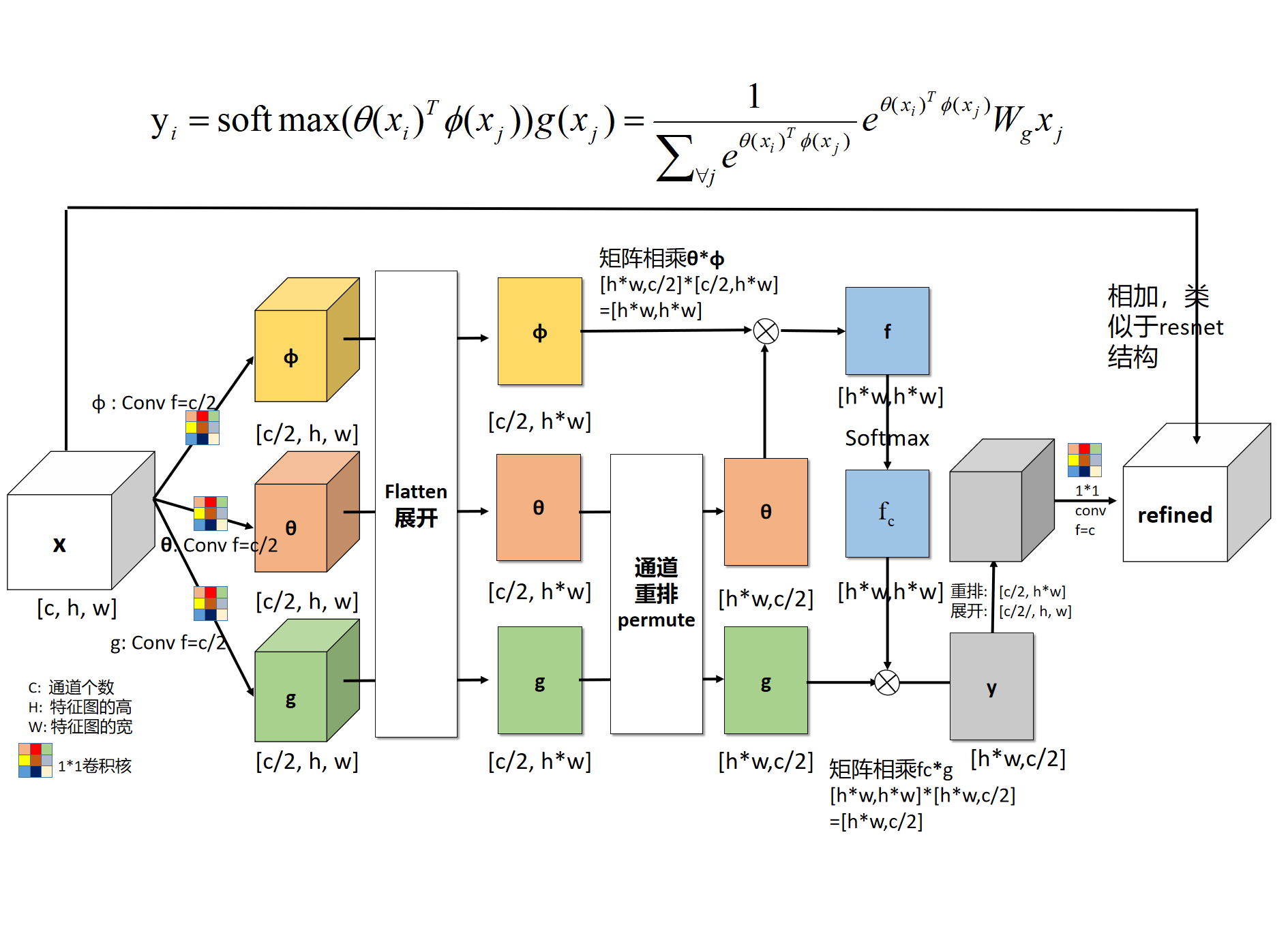• x代表feature map, $x_i$代表的是当前关注位置的信息； $x_j$代表的是全局信息。
• θ代表的是$\theta (x_i)=W_{\theta}x_i$ ,实际操作是用一个1×1卷积进行学习的。
• φ代表的是$\phi (x_j)=W_{\phi}x_j$,实际操作是用一个1×1卷积进行学习的。

• g函数意义同上。

• C(x)代表的是归一化操作，在embedding gaussian中使用的是Sigmoid实现的。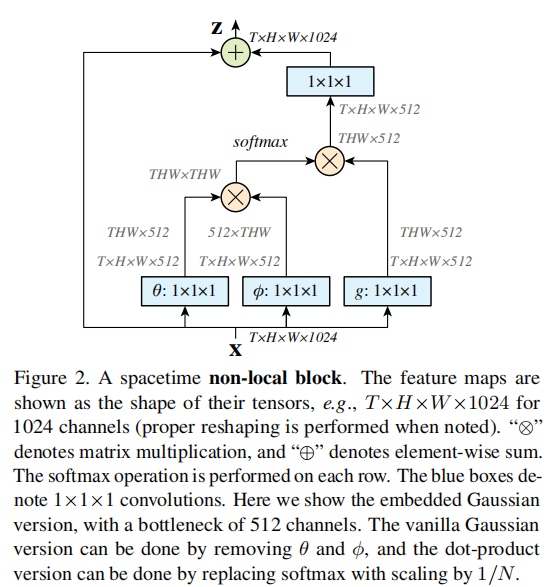X是一个feature map,形状为[bs, c, h, w], 经过三个1×1卷积核，将通道缩减为原来一半（c/2）。然后将h,w两个维度进行flatten，变为h×w，最终形状为[bs, c/2, h×w]的tensor。对θ对应的tensor进行通道重排，在线性代数中也就是转置，得到形状为[bs, h×w, c/2]。然后与φ代表的tensor进行矩阵乘法，得到一个形状为[bs, h×w，h×w]的矩阵，这个矩阵计算的是相似度（或者理解为attention）。然后经过softmax进行归一化，然后将该得到的矩阵$f_c$ 与g 经过flatten和转置的结果进行矩阵相乘，得到的形状为[bs, h*w, c/2]的结果y。然后转置为[bs, c/2, h×w]的tensor, 然后将h×w维度重新伸展为[h, w]，从而得到了形状为[bs, c/2, h, w]的tensor。然后对这个tensor再使用一个1×1卷积核，将通道扩展为原来的c，这样得到了[bs, c, h, w]的tensor,与初始X的形状是一致的。最终一步操作是将X与得到的tensor进行相加(类似resnet中的residual block)。

## 3. 代码¶

import torch
from torch import nn
from torch.nn import functional as F

class _NonLocalBlockND(nn.Module):
"""
调用过程
NONLocalBlock2D(in_channels=32),
super(NONLocalBlock2D, self).__init__(in_channels,
inter_channels=inter_channels,
dimension=2, sub_sample=sub_sample,
bn_layer=bn_layer)
"""
def __init__(self,
in_channels,
inter_channels=None,
dimension=3,
sub_sample=True,
bn_layer=True):
super(_NonLocalBlockND, self).__init__()

assert dimension in [1, 2, 3]

self.dimension = dimension
self.sub_sample = sub_sample

self.in_channels = in_channels
self.inter_channels = inter_channels

if self.inter_channels is None:
self.inter_channels = in_channels // 2
# 进行压缩得到channel个数
if self.inter_channels == 0:
self.inter_channels = 1

if dimension == 3:
conv_nd = nn.Conv3d
max_pool_layer = nn.MaxPool3d(kernel_size=(1, 2, 2))
bn = nn.BatchNorm3d
elif dimension == 2:
conv_nd = nn.Conv2d
max_pool_layer = nn.MaxPool2d(kernel_size=(2, 2))
bn = nn.BatchNorm2d
else:
conv_nd = nn.Conv1d
max_pool_layer = nn.MaxPool1d(kernel_size=(2))
bn = nn.BatchNorm1d

self.g = conv_nd(in_channels=self.in_channels,
out_channels=self.inter_channels,
kernel_size=1,
stride=1,

if bn_layer:
self.W = nn.Sequential(
conv_nd(in_channels=self.inter_channels,
out_channels=self.in_channels,
kernel_size=1,
stride=1,
nn.init.constant_(self.W.weight, 0)
nn.init.constant_(self.W.bias, 0)
else:
self.W = conv_nd(in_channels=self.inter_channels,
out_channels=self.in_channels,
kernel_size=1,
stride=1,
nn.init.constant_(self.W.weight, 0)
nn.init.constant_(self.W.bias, 0)

self.theta = conv_nd(in_channels=self.in_channels,
out_channels=self.inter_channels,
kernel_size=1,
stride=1,
self.phi = conv_nd(in_channels=self.in_channels,
out_channels=self.inter_channels,
kernel_size=1,
stride=1,

if sub_sample:
self.g = nn.Sequential(self.g, max_pool_layer)
self.phi = nn.Sequential(self.phi, max_pool_layer)

def forward(self, x):
'''
:param x: (b, c,  h, w)
:return:
'''

batch_size = x.size(0)

g_x = self.g(x).view(batch_size, self.inter_channels, -1)#[bs, c, w*h]
g_x = g_x.permute(0, 2, 1)

theta_x = self.theta(x).view(batch_size, self.inter_channels, -1)
theta_x = theta_x.permute(0, 2, 1)

phi_x = self.phi(x).view(batch_size, self.inter_channels, -1)

f = torch.matmul(theta_x, phi_x)

print(f.shape)

f_div_C = F.softmax(f, dim=-1)

y = torch.matmul(f_div_C, g_x)
y = y.permute(0, 2, 1).contiguous()
y = y.view(batch_size, self.inter_channels, *x.size()[2:])
W_y = self.W(y)
z = W_y + x
return z


## 4. 实验结论¶

• 文中提出了四个计算相似度的模型，实验对四个方法都进行了实验，发现了这四个模型效果相差并不大，于是有一个结论：使用non-local对baseline结果是有提升的，但是不同相似度计算方法之间差距并不大，所以可以采用其中一个做实验即可，文中用embedding gaussian作为默认的相似度计算方法。

• 作者做了一系列消融实验来证明non local NN的有效性：

1. 使用四个相似度计算模型，发现影响不大，但是都比baseline效果好。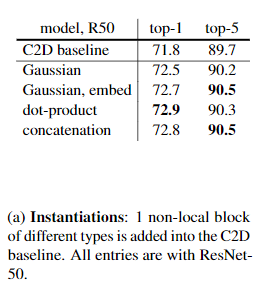2. 以ResNet50为例，测试加在不同stage下的结果。可以看出在res2,3,4部分得到的结果相对baseline提升比较大，但是res5就一般了，这有可能是由于第5个stage中的feature map的spatial size比较小，信息比较少，所以提升比较小。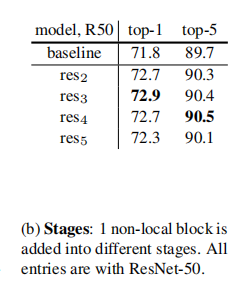3. 尝试添加不同数量的non local block ,结果如下。可以发现，添加越多的non local 模块，其效果越好，但是与此同时带来的计算量也会比较大，所以要对速度和精度进行权衡。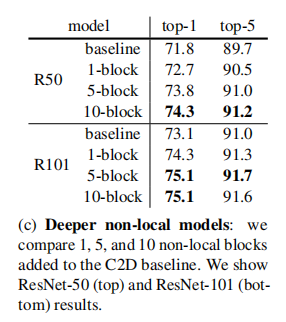4. Non-local 与3D卷积的对比，发现要比3D卷积计算量小的情况下，准确率有较为可观的提升。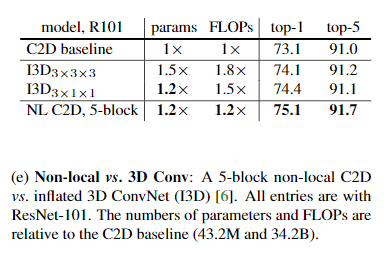5. 作者还将Non-local block应用在目标检测、实例分割、关键点检测等领域。可以将non-local block作为一个trick添加到目标检测、实例分割、关键点检测等领域, 可能带来1-3%的提升。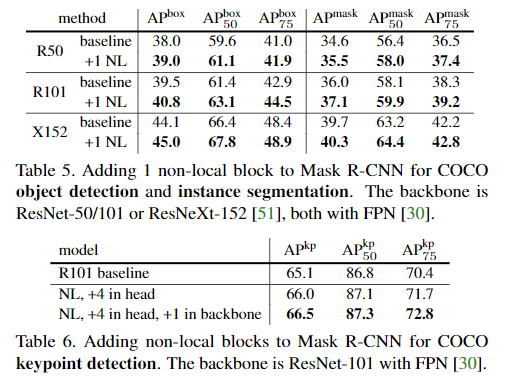## 5. 评价¶

Non local NN从传统方法Non local means中获得灵感，然后接着在神经网络中应用了这个思想，直接融合了全局的信息，而不仅仅是通过堆叠多个卷积层获得较为全局的信息。这样可以为后边的层带来更为丰富的语义信息。

## 6. 参考内容¶

CV中的Attention机制-最简单最易实现的SE模块

CV中的Attention机制-Selective-Kernel-Networks-SE进化版

CV中的Attention机制-CBAM模块

CV中的Attention机制-并行版的CBAM-BAM模块

CV中的attention机制-语义分割中的scSE模块